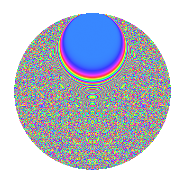# Properties

 Label 547.2.mLevel 547 Weight 2 Character orbit m Rep. character $$\chi_{547}(10,\cdot)$$ Character field $$\Q(\zeta_{91})$$ Dimension 3168 Newform subspaces 1 Sturm bound 91 Trace bound 0

# Related objects

## Defining parameters

 Level: $$N$$ $$=$$ $$547$$ Weight: $$k$$ $$=$$ $$2$$ Character orbit: $$[\chi]$$ $$=$$ 547.m (of order $$91$$ and degree $$72$$) Character conductor: $$\operatorname{cond}(\chi)$$ $$=$$ $$547$$ Character field: $$\Q(\zeta_{91})$$ Newform subspaces: $$1$$ Sturm bound: $$91$$ Trace bound: $$0$$

## Dimensions

The following table gives the dimensions of various subspaces of $$M_{2}(547, [\chi])$$.

Total New Old
Modular forms 3312 3312 0
Cusp forms 3168 3168 0
Eisenstein series 144 144 0

## Trace form

 $$3168q - 76q^{2} - 38q^{3} - 34q^{4} - 75q^{5} - 42q^{6} - 60q^{7} - 77q^{8} - 550q^{9} + O(q^{10})$$ $$3168q - 76q^{2} - 38q^{3} - 34q^{4} - 75q^{5} - 42q^{6} - 60q^{7} - 77q^{8} - 550q^{9} - 91q^{10} - 61q^{11} - 31q^{12} - 72q^{13} - 21q^{14} - 46q^{15} + 24q^{16} - 91q^{17} + 9q^{18} - 89q^{19} - 108q^{20} - 81q^{21} - 50q^{22} - 86q^{23} - 33q^{24} - 15q^{25} + 137q^{26} + 163q^{27} - 47q^{28} - 12q^{29} + 29q^{30} - 53q^{31} - 122q^{32} - 21q^{33} - 80q^{34} - 75q^{35} - 8q^{36} - 49q^{37} - 47q^{38} + 128q^{39} - 246q^{40} - 686q^{41} - 466q^{42} - 9q^{43} - 43q^{44} + 156q^{45} - 91q^{46} - 72q^{47} + 21q^{48} - 8q^{49} - 84q^{50} + 93q^{51} + 4q^{52} - 32q^{53} - 71q^{54} - 34q^{55} - 263q^{56} - 61q^{57} - 36q^{58} - 23q^{59} + 87q^{60} + 38q^{61} + 26q^{62} + 133q^{63} + 343q^{64} + 143q^{65} - 65q^{66} - 50q^{67} - 213q^{68} - 140q^{69} + 372q^{70} - 75q^{71} + 147q^{72} - 18q^{73} - 85q^{74} + 97q^{75} - 47q^{76} + 11q^{77} + 55q^{78} - 37q^{79} - 142q^{80} - 532q^{81} - 94q^{82} - 279q^{83} + 395q^{84} - 75q^{85} - 147q^{86} + 84q^{87} + 345q^{88} + 8q^{89} + 278q^{90} - 38q^{91} - 78q^{92} - 83q^{93} - 540q^{94} + 35q^{95} + 123q^{96} - 735q^{97} - 90q^{98} + 351q^{99} + O(q^{100})$$

## Decomposition of $$S_{2}^{\mathrm{new}}(547, [\chi])$$ into newform subspaces

Label Dim. $$A$$ Field CM Traces $q$-expansion
$$a_2$$ $$a_3$$ $$a_5$$ $$a_7$$
547.2.m.a $$3168$$ $$4.368$$ None $$-76$$ $$-38$$ $$-75$$ $$-60$$

## Hecke characteristic polynomials

There are no characteristic polynomials of Hecke operators in the database